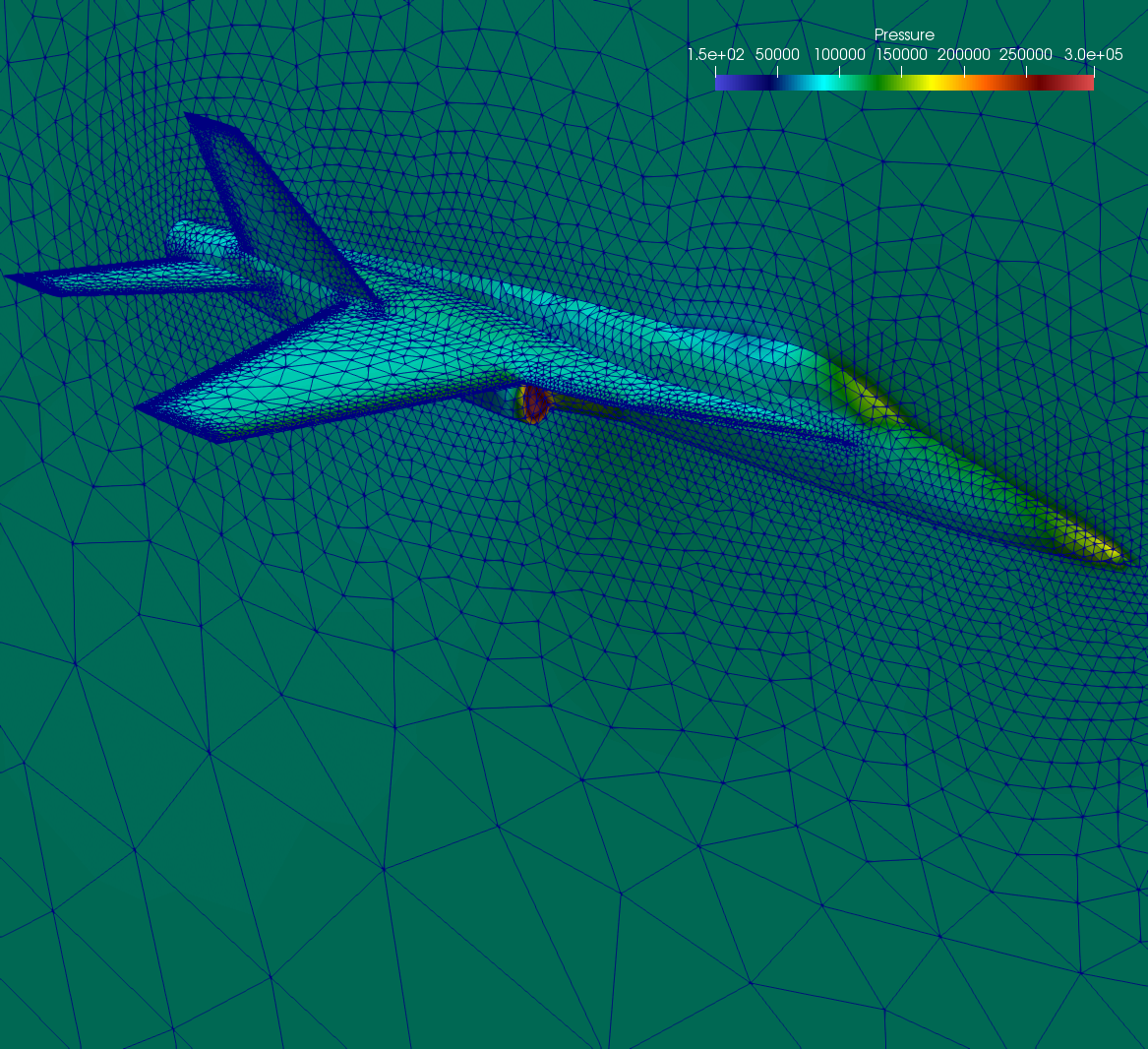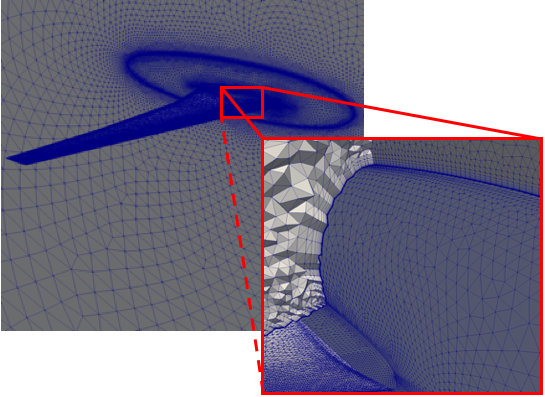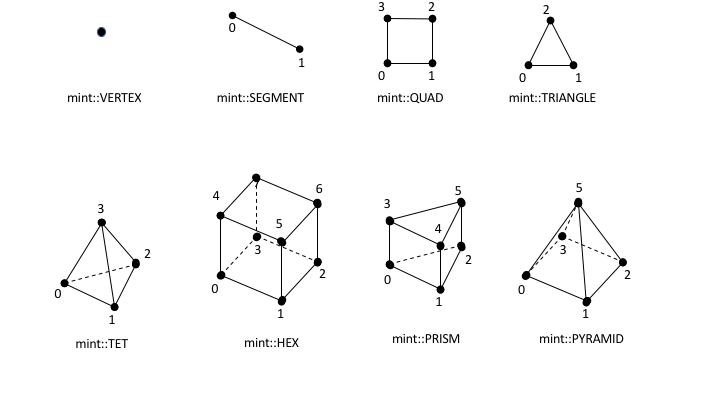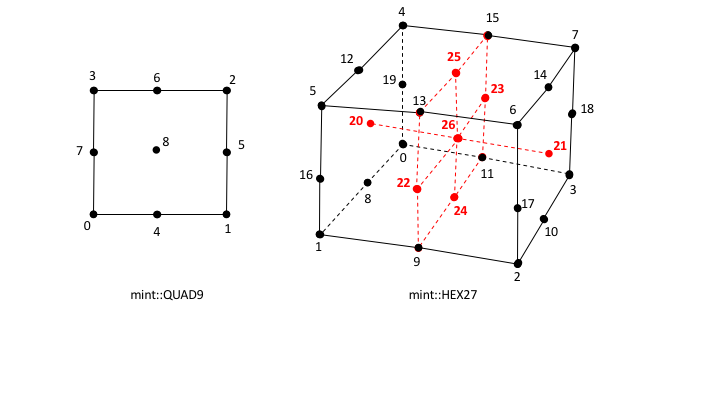# Mesh Types¶

The underlying, concrete, representation of the constituent Geometry and Topology of a mesh is the key defining charachteristic used in classifying a mesh into the different Mesh Types. The Geometry and Topology of a mesh is specified in one of the following three representations:

1. Implicit Representation: based on mesh metadata
2. Explicit Representation: employs explicitly stored information.
3. Semi-Implicit Representation: combines mesh metadata and explicitly stored information.

The possible representation combinations of the constituent Geometry and Topology comprising a mesh define a taxonomy of Mesh Types summarized in the table below.

Mesh Type Geometry Topology
Curvilinear Mesh explicit implicit
Rectilinear Mesh semi-implicit implicit
Uniform Mesh implicit implicit
Unstructured Mesh explicit explicit
Particle Mesh explicit implicit

A brief overview of the distinct characteristics of each of the Mesh Types is provided in the following sections.

## Structured Mesh¶

A Structured Mesh discretization is characterized by its ordered, regular, Topology. A Structured Mesh divides the computational domain into Cells that are logically arranged on a regular grid. The regular grid topology allows for the constituent Nodes, Cells and Faces of the mesh to be identified using an IJK ordering scheme.

Numbering and Ordering Conventions in a Structured Mesh

The IJK ordering scheme employs indices along each dimension, typically using the letters i,j,k for the 1st, 2nd and 3rd dimension respectively. The IJK indices can be thought of as counters. Each index counts the number of Nodes or Cells along a given dimension. As noted in the general Mesh Representation section, the constituent entities of the mesh Topology are associated with a unique index. Therefore, a convention needs to be established for mapping the IJK indices to the corresponding unique index and vice-versa.

The general convention and what Mint employs is the following:

• All Nodes and Cells of a Structured Mesh are indexed first along the I-direction, then along the J-direction and last along the K-direction.
• Likewise, the Faces of a Structured Mesh are indexed by first counting the Faces of each of the Cells along the I-direction (I-Faces), then the J-direction (J-Faces) and last the K-direction (K-Faces).

One of the important advantages of a Structured Mesh representation is that the constituent Topology of the mesh is implicit. This enables a convenient way for computing the Connectivity information automatically without the need to store this information explicitly. For example, an interior 2D cell (i.e., not at a boundary) located at $$C=(i,j)$$, will always have four face neighbors given by the following indices:

• $$N_0=(i-1,j)$$,
• $$N_1=(i+1,j)$$,
• $$N_2=(i,j-1)$$ and
• $$N_3=(i,j+1)$$

Notably, the neighboring information follows directly from the IJK ordering scheme and therefore does not need to be stored explicitly.

In addition to the convenience of having automatic Connectivity, the IJK ordering of a Structured Mesh offers one other important advantage over an Unstructured Mesh discretization. The IJK ordering results in coefficient matrices that are banded. This enables the use of specialized algebraic solvers that rely on the banded structure of the matrix that are generally more efficient.

While a Structured Mesh discretization offers several advantages, there are some notable tradeoffs and considerations. Chief among them, is the implied restriction imposed by the regular topology of the Structured Mesh. Namely, the number of Nodes and Cells on opposite sides must be matching. This requirement makes local refinement less effective, since grid lines need to span across the entire range along a given dimension. Moreover, meshing of complex geometries, consisting of sharp features, is complicated and can lead to degenerate Cells that can be problematic in the computation. These shortcomings are alleviated to an extent using a block-structured meshing strategy and/or patch-based AMR, however the fundamental limitations still persist.

All Structured Mesh types have implicit Topology. However, depending on the underlying, concrete representation of the consituent mesh Geometry, a Structured Mesh is distinguished into three subtypes:

The key characteristics of each of theses types is discussed in more detail in the following sections.

### Curvilinear Mesh¶

The Curvilinear Mesh, shown in Fig. 8, is logically a regular mesh, however in contrast to the Rectilinear Mesh and Uniform Mesh, the Nodes of a Curvilinear Mesh are not placed along the Cartesian grid lines. Instead, the equations of the governing PDE are transformed from the Cartesian coordinates to a new coordinate system, called a curvilinear coordinate system. Consequently, the Topology of a Curvilinear Mesh is implicit, however its Geometry, given by the constituent Nodes of the mesh, is explicit.

The mapping of coordinates to the curvilinear coordinate system facilitates the use of structured meshes for bodies of arbitrary shape. Note, the axes defining the curvilinear coordinate system do not need to be straight lines. They can be curves and align with the contours of a solid body. For this reason, the resulting Curvilinear Mesh is often called a mapped mesh or body-fitted mesh.

See the Tutorial for an example that demonstrates how to Create a Curvilinear Mesh.

### Rectilinear Mesh¶

A Rectilinear Mesh, depicted in Fig. 9, divides the computational domain into a set of rectangular Cells, arranged on a regular lattice. However, in contrast to the Curvilinear Mesh, the Geometry of the mesh is not mapped to a different coordinate system. Instead, the rows and columns of Nodes comprising a Rectilinear Mesh are parallel to the axis of the Cartesian coordinate system. Due to this restriction, the geometric domain and resulting mesh are always rectangular.

The Topology of a Rectilinear Mesh is implicit, however its constituent Geometry is semi-implicit. Although, the Nodes are aligned with the Cartesian coordinate axis, the spacing between adjacent Nodes can vary. This allows a Rectilinear Mesh to have tighter spacing over regions of interest and be sufficiently coarse in other parts of the domain. Consequently, the spatial coordinates of the Nodes along each axis are specified explicitly in a seperate array for each coordinate axis, i.e. $$x$$, $$y$$ and $$z$$ arrays for each dimension respectively. Given the IJK index of a node, its corresponding physical coordinates can be obtained by taking the Cartesian product of the corresponding coordinate along each coordinate axis. For this reason, the Rectilinear Mesh is sometimes called a product mesh.

See the Tutorial for an example that demonstrates how to Create a Rectilinear Mesh.

### Uniform Mesh¶

A Uniform Mesh, depicted in Fig. 10, is the simplest of all three Structured Mesh types, but also, relatively the most restrictive of all Mesh Types. As with the Rectilinear Mesh, a Uniform Mesh divides the computational domain into a set of rectangular Cells arranged on a regular lattice. However, a Uniform Mesh imposes the additional restriction that Nodes are uniformly distributed parallel to each axis. Therefore, in contrast to the Rectilinear Mesh, the spacing between adjacent Nodes in a Uniform Mesh is constant.

The inherent constraints of a Uniform Mesh allow for a more compact representation. Notably, both the Topology and Geometry of a Uniform Mesh are implicit. Given the origin of the mesh, $$X_0=(x_0,y_0,z_0)^T$$, i.e. the coordinates of the lowest corner of the rectangular domain, and spacing along each direction, $$H=(h_x,h_y,h_z)^T$$, the spatial coordinates of any point, $$\hat{p}=(p_x,p_y,p_z)^T$$, corresponding to a node with lattice coordinates, $$(i,j,k)$$, are computed as follows:

\begin{eqnarray} p_x &=& x_0 &+& i &\times& h_x \\ p_y &=& y_0 &+& j &\times& h_y \\ p_z &=& z_0 &+& k &\times& h_z \\ \end{eqnarray}

See the Tutorial for an example that demonstrates how to Create a Uniform Mesh.

## Unstructured Mesh¶

The impetus for an Unstructured Mesh discretization is largely prompted by the need to model physical phenomena on complex geometries. In relation to the various Mesh Types, an Unstructured Mesh discretization provides the most flexibility. Notably, an Unstructured Mesh can accomodate different Cell Types and does not enforce any constraints or particular ordering on the constituent Nodes and Cells. This makes an Unstructured Mesh discretization particularly attractive, especially for applications that require local adaptive mesh refinement (i.e., local h-refinement) and deal with complex geometries.

Generally, the advantages of using an Unstructured Mesh come at the cost of an increase in memory requirements and computational intensity. This is due to the inherently explicit, Mesh Representation required for an Unstructured Mesh. Notably, both Topology and Geometry are represented explicitly thereby increasing the storage requirements and computational time needed per operation. For example, consider a stencil operation. For a Structured Mesh, the neighbor indices needed by the stencil can be automatically computed directly from the IJK ordering, a relatively fast and local operation. However, to obtain the neighbor indices in an Unstructured Mesh, the arrays that store the associated Connectivity information need to be accessed, resulting in additional load/store operations that are generaly slower.

Depending on the application, the constituent Topology of an Unstructured Mesh may employ a:

1. Single Cell Type Topology, i.e. consisting of Cells of the same type, or,
2. Mixed Cell Type Topology, i.e. consisting of Cells of different type, i.e. mixed cell type.

There are subtle differrences in the underlying Mesh Representation that can result in a more compact and efficient representation when the Unstructured Mesh employs a Single Cell Type Topology. The following sections discuss briefly these differences and other key aspects of the Single Cell Type Topology and Mixed Cell Type Topology representations. Moreover, the list of natively supported Cell Types that can be used with an Unstructured Mesh is presented, as well as, the steps necessary to Add a New Cell Type in Mint.

Note

In an effort to balance both flexibility and simplicity, Mint, in its simplest form, employs the minumum sufficient Unstructured Mesh Mesh Representation, consisting of the cell-to-node Connectivity. This allows applications to employ a fairly light-weight mesh representation when possible. However, for applications that demand additional Connectivity information, Mint provides methods to compute the needed additional information.

### Single Cell Type Topology¶

An Unstructured Mesh with Single Cell Type Topology consists of a collection of Cells of the same cell type. Any Structured Mesh can be treated as an Unstructured Mesh with Single Cell Type Topology, in which case, the resulting Cells would either be segments (in 1D), quadrilaterals (in 2D) or hexahedrons (in 3D). However, an Unstructured Mesh can have arbitrary Connectivity and does not impose any ordering constraints. Moreover, the Cells can also be triangular (in 2D) or tetrahedral (in 3D). The choice of cell type generally depends on the application, the physics being modeled, and the numerical scheme employed. An example tetrahedral Unstructured Mesh of the F-17 blended wing fuselage configuration is shown in Fig. 11. For this type of complex geometries it is nearly impossible to obtain a Structured Mesh that is adequate for computation.Fig. 11 Sample unstructured tetrahedral mesh of the F-17 blended wing fuselage configuration.

Mint’s Mesh Representation of an Unstructured Mesh with Single Cell Type Topology consists of a the cell type specification and the cell-to-node Connectivity information. The Connectivity information is specified with a flat array consisting of the node indices that comprise each cell. Since the constituent mesh Cells are of the same type, cell-to-node information for a particular cell can be obtained by accessing the Connectivity array with a constant stride, where the stride corresponds to the number of Nodes of the cell type being used. This is equivalent to a 2D row-major array layout where the number of rows corresponds to the number of Cells in the mesh and the number of columns corresponds to the stride, i.e. the number of Nodes per cell.Fig. 12 Mesh Representation of an Unstructured Mesh with Single Cell Type Topology consiting of triangular Cells. Knowing the cell type enables traversing the cell-to-node Connectivity array with a constant stride of $$3$$, which corresponds to the number of constituent Nodes of each triangle.

This simple concept is best illustrated with an example. Fig. 12 depicts a sample Unstructured Mesh with Single Cell Type Topology consisting of $$N_c=4$$ triangular Cells. Each triangular cell, $$C_i$$, is defined by $$||C_i||$$ Nodes. In this case, $$||C_i||=3$$.

Note

The number of Nodes of the cell type used to define an Unstructured Mesh with Single Cell Type Topology, denoted by $$||C_i||$$, corresponds to the constant stride used to access the flat cell-to-node Connectivity array.

Consequently, the length of the cell-to-node Connectivity array is then given by $$N_c \times ||C_i||$$. The node indices for each of the cells are stored from left to right. The base offset for a given cell is given as a multiple of the cell index and the stride. As illustrated in Fig. 12, the base offset for cell $$C_0$$ is $$0 \times 3 = 0$$, the offest for cell $$C_1$$ is $$1 \times 3 = 3$$, the offset for cell $$C_2$$ is $$2 \times 3 = 6$$ and so on.

Direct Stride Cell Access in a Single Cell Type Topology UnstructuredMesh

In general, the Nodes of a cell, $$C_i$$, of an Unstructured Mesh with Single Cell Type Topology and cell stride $$||C_i||=k$$, can be obtained from a given cell-to-node Connectivity array as follows:

\begin{eqnarray} n_0 &=& cell\_to\_node[ i \times k ] \\ n_1 &=& cell\_to\_node[ i \times k + 1 ] \\ ... \\ n_k &=& cell\_to\_node[ i \times k + (k-1)] \end{eqnarray}
Cell Type Stride Topological Dimension Spatial Dimension
Triangle 3 2 2,3
Hexahdron 8 3 3
Tetrahedron 4 3 3

The same procedure follows for any cell type. Thereby, the stride for a mesh consisting of quadrilaterals is $$4$$, the stride for a mesh consisting of tetrahedrons is $$4$$ and the stride for a mesh consisting of hexahedrons is $$8$$. The table above summarizes the possible Cell Types that can be employed for an Unstructured Mesh with Single Cell Type Topology, corresponding stride and applicalble topological and spatial dimension.

See the Tutorial for an example that demonstrates how to Create an Unstructured Mesh.

### Mixed Cell Type Topology¶

An Unstructured Mesh with Mixed Cell Type Topology provides the most flexibility relative to the other Mesh Types. Similar to the Single Cell Type Topology Unstructured Mesh, the constituent Nodes and Cells of a Mixed Cell Type Topology Unstructured Mesh can have arbitrary ordering. Both Topology and Geometry are explicit. However, a Mixed Cell Type Topology Unstructured Mesh may consist Cells of different cell type. Hence, the cell topology and cell type is said to be mixed.

Note

The constituent Cells of an Unstructured Mesh with Mixed Cell Type Topology have a mixed cell type. For this reason, an Unstructured Mesh with Mixed Cell Type Topology is sometimes also called a mixed cell mesh or hybrid mesh.Fig. 13 Sample Unstructured Mesh with Mixed Cell Type Topology of a Generic wing/fuselage configuration. The mesh consists of high-aspect ratio prism cells in the viscous region of the computational domain to accurately capture the high gradients across the boundary layer and tetrahedra cells for the inviscid/Euler portion of the mesh.

Several factors need to be taken in to account when selecting the cell topology of the mesh. The physics being modeled, the PDE discretization employed and the required simulation fidelity are chief among them. Different Cell Types can have superior properties for certain calculations. The continuous demand for increasing fidelity in physics-based predictive modeling applications has prompted practitioners to employ a Mixed Cell Type Topology Unstructured Mesh discretization in order to accurately capture the underlying physical phenomena.

For example, for Navier-Stokes viscous fluid-flow computations, at high Reynolds numbers, it is imperative to capture the high gradients across the boundary layer normal to the wall. Typically, high-aspect ratio, anisotropic triangular prisms or hexahedron Cells are used for discretizing the viscous region of the computational domain, while isotropic tetrahedron or hexahedron Cells are used in the inviscid region to solve the Euler equations. The sample Mixed Cell Type Topology Unstructured Mesh, of a Generic Wing/Fuselage configuration, depicted in Fig. 13, consists of triangular prism Cells for the viscous boundary layer portion of the domain that are stitched to tetrahedra Cells for the inviscid/Euler portion of the mesh.

The added flexibility enabled by employing a Mixed Cell Type Topology Unstructured Mesh imposes additional requirements to the underlying Mesh Representation. Most notably, compared to the Single Cell Type Topology Mesh Representation, the cell-to-node Connectivity array can consist Cells of different cell type, where each cell can have a different number of Nodes. Consequently, the simple stride array access indexing scheme, used for the Single Cell Type Topology Mesh Representation, cannot be employed to obtain cell-to-node information. For a Mixed Cell Type Topology an indirect addressing access scheme must be used instead.Fig. 14 Mesh Representation of a Mixed Cell Type Topology Unstructured Mesh with a total of $$N=3$$ Cells, $$2$$ triangles and $$1$$ quadrilateral. The Mixed Cell Type Topology representation consists of two additional arrays. First, the Cell Offsets array, an array of size $$N+1$$, where the first $$N$$ entries store the starting position to the flat cell-to-node Connectivity array for each cell. The last entry of the Cell Offsets array stores the total length of the Connectivity array. Second, the Cell Types array , an array of size $$N$$, which stores the cell type of each constituent cell of the mesh.

There are a number of ways to represent a Mixed Cell Type Topology mesh. In addition to the cell-to-node Connectivity array, Mint’s Mesh Representation for a Mixed Cell Type Topology Unstructured Mesh employs two additional arrays. See sample mesh and corresponding Mesh Representation in Fig. 14. First, the Cell Offsets array is used to provide indirect addressing to the cell-to-node information of each constituent mesh cell. Second, the Cell Types array is used to store the cell type of each cell in the mesh.

The Cell Offsets is an array of size $$N+1$$, where the first $$N$$ entries, corresponding to each cell in the mesh, store the start index position to the cell-to-node Connectivity array for the given cell. The last entry of the Cell Offsets array stores the total length of the Connectivity array. Moreover, the number of constituent cell Nodes for a given cell can be directly computed by subtracting a Cell’s start index from the next adjacent entry in the Cell Offsets array.

However, knowing the start index position to the cell-to-node Connectivity array and number of constituent Nodes for a given cell is not sufficient to disambiguate and deduce the cell type. For example, both tetrahedron and quadrilateral Cells are defined by $$4$$ Nodes. The cell type is needed in order to correctly interpret the Topology of the cell according to the cell’s local numbering. Consequently, the Cell Types array, whose length is $$N$$, corresponding to the number of cells in the mesh, is used to store the cell type for each constituent mesh cell.

Indirect Address Cell Access in a Mixed Cell Type Topology UnstructuredMesh

In general, for a given cell, $$C_i$$, of a Mixed Cell Type Topology Unstructured Mesh, the number of Nodes that define the cell, $$||C_i||$$, is given by:

\begin{eqnarray} k = ||C_i|| &=& cells\_offset[ i + 1 ] - cells\_offset[ i ] \\ \end{eqnarray}

The corresponding cell type is directly obtained from the Cell Types array:

\begin{eqnarray} ctype &=& cell\_types[ i ] \\ \end{eqnarray}

The list of constituent cell Nodes can then obtained from the cell-to-node Connectivity array as follows:

\begin{eqnarray} offset &=& cells\_offset[ i+1 ] \\ k &=& cells\_offset[ i + 1 ] - cell\_offset[ i ] \\ \\ n_0 &=& cell\_to\_node[ offset ] \\ n_1 &=& cell\_to\_node[ offset + 1 ] \\ ... \\ n_k &=& cell\_to\_node[ offset + (k-1)] \end{eqnarray}

See the Tutorial for an example that demonstrates how to Create a Mixed Unstructured Mesh.

### Cell Types¶

Mint currently supports the common Linear Cell Types, depicted in Fig. 15, as well as, support for quadratic, quadrilateral and hexahedron Cells, see Fig. 16.Fig. 15 List of supported linear cell types and their respective local node numbering.Fig. 16 List of supported quadratic cell types and their respective local node numbering.

Note

All Mint Cell Types follow the CGNS Numbering Conventions.

Moreover, Mint is designed to be extensible. It is relatively straightforward to Add a New Cell Type in Mint. Each of the Cell Types in Mint simply encode the following attributes:

• the cell’s topology, e.g. number of nodes, faces, local node numbering etc.,
• the corresponding VTK type, used for VTK dumps, and,
• the associated blueprint name, conforming to the Blueprint conventions, used for storing the mesh in Sidre

Warning

The Blueprint specification does not currently support the following cell types:

1. Transitional cell types, Pyramid(mint::PYRAMID) and Prism(mint::PRISM)
2. Quadratic cells, the 9-node, quadratic Quadrilateral(mint::QUAD9) and the 27-node, quadratic Hexahedron(mint::HEX27)

### Add a New Cell Type¶

Warning

This section is under construction.

## Particle Mesh¶

A Particle Mesh, depicted in Fig. 17, discretizes the computational domain by a set of particles which correspond to the Nodes at which the solution is evaluated. A Particle Mesh is commonly employed in the so called particle methods, such as Smoothed Particle Hydrodynamics (SPH) and Particle-In-Cell (PIC) methods, which are used in a variety of applications ranging from astrophysics and cosmology simulations to plasma physics.

There is no special ordering imposed on the particles. Therefore, the particle coordinates are explicitly specified by nodal coordinates, similar to an Unstructured Mesh. However, the particles are not connected to form a control volume, i.e. a filled region of space. Consequently, a Particle Mesh does not have Faces and any associated Connectivity information. For this reason, methods that employ a Particle Mesh discretization are often referred to as meshless or mesh-free methods.

A Particle Mesh can be thought of as having explicit Geometry, but, implicit Topology. Mint’s Mesh Representation for a Particle Mesh, associates the constituent particles with the Nodes of the mesh. The Nodes of the Particle Mesh can also be thought of as Cells that are defined by a single node index. However, since this information can be trivially obtained there is no need to be stored explicitly.

Note

A Particle Mesh can only store variables at its constituent particles, i.e. the Nodes of the mesh. Consequently, a Particle Mesh in Mint can only be associated with node-centered Field Data.